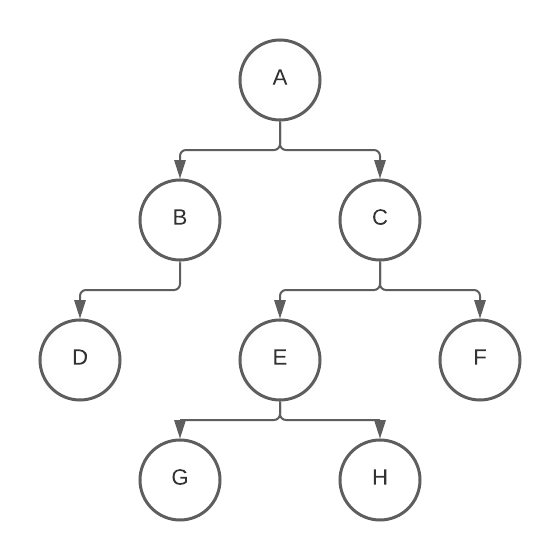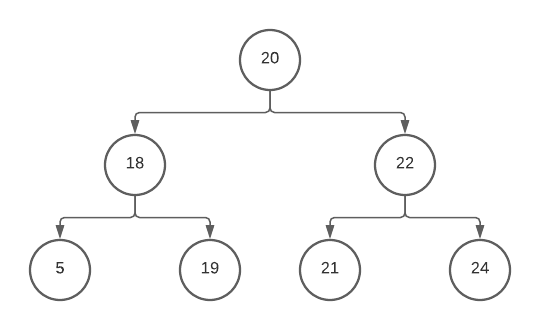# Implement Tree Data Structure in Python

In the data structure, a tree is a type of nonlinear data structure that consists of nodes that are connected. A tree typically has a single root node which indicates the starting point of the data structure.

Trees are one of the most challenging topics to learn in data structures and programming. Application-wise, trees are typically used for efficient data storage and fast traversal and indexing when searching for data.

This tutorial will demonstrate how to implement the tree data structure in Python. For this tutorial, we will be focusing on implementing binary trees.

Binary trees are the easiest to remember and implement, so this will be the main focus of this tutorial.

## Implement a Tree Class Manually in Python

Python isn’t exactly known for being object-oriented and does not support data structures as much as other languages that focus on object creation.

Since Python supports the creation and instantiation of classes, implement trees by creating a class `Tree` and define the fields. An instance of data in a tree is called a `node`. Trees are composed of nodes, having a single root node that can span indefinitely.

Binary Trees are the most common structure of trees. The primary distinction that a tree is a binary tree is that there can only be at most two children nodes per parent node.

Here’s a visual representation of how a binary tree might look like.In the visual representation of a tree above, `A` is the root node. Observe that each node can only have at most two children or no children at all.

### Declare a Tree

To declare a binary tree in Python, create a class `Tree` with an `__init__()` function that will instantiate these three class fields: the left child node, the right child node, and the data of the current node. The three fields mentioned is the composition of a simple binary tree.

``````class Tree:
def __init__(self):
self.val = None
self.left = None
self.right = None
``````

The `__init__()` function is Python’s version of a class constructor in OOP. This is the function called when an instance of the `Tree` class is created. In this case, it initially sets the value and the children nodes to `None`.

Another approach to declare a tree in Python is to optionally include the value of a tree within the constructor. To do this, add a second parameter to the `__init__()` function representing the value of the tree and initialize it to `None` to make it an optional parameter.

``````class Tree:
def __init__(self, val = None):
if val != None:
self.val = val
else:
self.val = None

self.left = None
self.right = None
``````

This approach allows having the value of the tree instantiated together with the actual tree and at the same time setting it to `None` if there is no `val` argument.

### Create an Instance of a Tree

Now that the declaration of a binary tree is covered, we can now instantiate an instance of a tree.

To do this, we just call the constructor of the object by using the name of the object. In this case, it would be `Tree()` since the `__init__()` function does not hold any arguments except for itself.

For example, to instantiate a tree without any arguments:

``````tree = Tree()

print(tree)
``````

Output:

``````<__main__.Tree object at 0x10cd98dd8>
``````

The output represents the area in memory that is allocated for the tree object that has just been instantiated.

To manually add a value to the tree, assign a value to the `val` object within the newly instantiated tree.

``````tree = Tree()
tree.val = 20
print(tree.val)
``````

Output:

``````20
``````

Using the alternative approach which accepts the `val` field as an argument will further shorten this operation.

``````tree = Tree(20)
print(tree.val)
``````

Output:

``````20
``````

Both approaches will perform the same action though the latter approach is considerably more efficient.

Now, to instantiate the children of the existing tree, just do the same thing above but to the `left` and `right` fields within the `tree` object.

``````tree = Tree(20)
tree.left = Tree(18)
tree.right = Tree(22)

print(tree.left.val)
print(tree.right.val)
``````

Output:

``````18
22
``````

If we illustrate this like the visual representation above, the tree would initially look like this:The main rule of a binary tree is that all the nodes within the tree are arranged in a specific order. This is done so that traversing a binary tree is supported with some sort of logic. In this case, the logic is that the tree contains integer values and is arranged in the ascending order from left to right.

Now, how do we go on about inserting a new element into the tree?

### Insert an Element to an Existing Tree

To insert an element into an existing tree, add a new function, `insert()`, into the `Tree` class. The function accepts two parameters: the self-referential parameter `self`, and the value to be inserted `val`.

The `insert()` function inserts the value `val` into the tree by traversing the tree to locate where the value should be inserted based on the given logic. Again, the logic for the example in this article is in ascending order based on the integer values.

This function is recursive by nature, which means that it can go up and down the tree depending on the logic declared. Recursive functions are functions that repeatedly call itself within the function until it reaches an exit condition.

``````def insert(self, val):
if self.val:
if val < self.val:
if self.left is None:
self.left = Tree(val)
else:
self.left.insert(val)
elif val > self.val:
if self.right is None:
self.right = Tree(val)
else:
self.right.insert(val)
else:
self.val = val
``````

The function above performs the following:

• If the current node value is empty, the function assigns `val` to the current node.
• If the current node value is greater than the value to be inserted, check if the current node has a left child
• If the left child exists, call the `insert()` function again, with the left child as the self-referential argument (recursive call).
• If the left child does not exist, assign `val` to the current node.
• If the current node value is lesser than the value to be inserted, check if the current node has a left child
• If the right child exists, call the `insert()` function again, with the right child as the self-referential argument (recursive call).
• If the right child does not exist, assign `val` to the current node.

Note that binary trees always will insert values and will never replace nor displace any existing values.

Now, with the existing example given in the last section, let’s try inserting the number `19` as a new value in the tree.

``````tree = Tree(20)
tree.left = Tree(18)
tree.right = Tree(22)
tree.insert(19)
``````

Ideally, if the function is implemented properly, the tree with the newly inserted value should look like this.So to print it out explicitly, it would be as below.

``````tree = Tree(20)
tree.left = Tree(18)
tree.right = Tree(22)
tree.insert(19)

print(tree.left.right)
print(tree.left.right.val)
``````

Output:

``````<__main__.Tree object at 0x109692fd0>
19
``````

Now, what if we want to print the entire contents of the tree in ascending order? A traversal function would also have to be implemented.

### Traverse the Entire Tree

To traverse a binary tree and print out the contents in the desired order, we should use the in-order traversal. This type of traversal starts printing out the values from the left, then to the center, and then finally to the right.

Tree traversal functions also have to be recursive.

Here is the code to traverse the existing tree from the example above:

``````def printValues(self):
if self.left:
self.left.printValues()

print(self.val)

if self.right:
self.right.printValues()

``````

Let’s test this function out with the existing example in the last section but with more inserted elements.

``````tree = Tree(20)
tree.left = Tree(18)
tree.right = Tree(22)
tree.insert(19)
tree.insert(24)
tree.insert(5)
tree.insert(21)

tree.printValues()
``````

Visually, the tree would look like this:And the output of the `printValues()` function would be:

``````5
18
19
20
21
22
24
``````

Expectedly, the output would display the contents of the tree in ascending order.

Here is the final compiled source code for the final example:

``````class Tree:
def __init__(self, val = None):
if val != None:
self.val = val
else:
self.val = None
self.left = None
self.right = None

def insert(self, val):
if self.val:
if val < self.val:
if self.left is None:
self.left = Tree(val)
else:
self.left.insert(val)
elif val > self.val:
if self.right is None:
self.right = Tree(val)
else:
self.right.insert(val)
else:
self.val = val

def printValues(self):
if self.left:
self.left.printValues()
print(self.val)
if self.right:
self.right.printValues()

tree = Tree(20)
tree.left = Tree(18)
tree.right = Tree(22)
tree.insert(19)
tree.insert(24)
tree.insert(5)
tree.insert(21)

tree.printValues()
``````

In summary, binary trees in Python are simple to implement and instantiate. You would have to manually create a tree object in Python and create the utility functions for insertion and traversal. Also, there should be a specific logic for the implementation and for recursive functions to have an exit condition. In the case of this tutorial, the logic implemented is integers arranged by ascending order.

Contribute
DelftStack is a collective effort contributed by software geeks like you. If you like the article and would like to contribute to DelftStack by writing paid articles, you can check the write for us page.